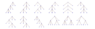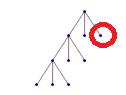# Upper bound height and lower bound height of a 3-ary ordered tree

• fiksx
What does it mean: not necessarily complete, but three children? At each level, always, once?For an m-ary tree with height h, the upper bound for the maximum number of leaves is {\displaystyle m^{h}}.The height h of an m-ary tree does not include the root node, with a tree containing only a root node having a height of 0.The height of a tree is equal to the maximum depth D of any node in the tree.The total number of nodes {\displaystyle N} in a perfect m-ary tree is {\textstyle \sum _{i=0}^{h}m^{i}={\frac {m^{h+f

#### fiksx

how to find upper bound height and lower bound height of 3-ary ordered tree that have leaves of 101? ( the tree don't have to be complete tree, but have to be have 3 children)
$$m^h \ge 101=3^h \ge 101$$

$$log \, m^h \ge 101=3^h \ge 101$$

$$h \ge 5$$

but how to know upper bound and lower bound of leaves?

edit: leaves at the last height is 3
$$3+2(n-1) \ge 101= 3+2n-2 \ge 101 = 2n-1 \ge 101= 2n \ge 100 = n \ge 50$$

is this right?

[Moderator's note: Moved from a technical forum and thus no template.]

Last edited by a moderator:
Can you give an example? What does it mean: not necessarily complete, but three children? At each level, always, once?

Upper bounds are easy to find: you only have to give an example.
Lower bounds are the difficult part: you have to find a height which is provable needed at least. For this we need a very accurate definition on all properties of the tree.

Can you give an example? What does it mean: not necessarily complete, but three children? At each level, always, once?

Upper bounds are easy to find: you only have to give an example.
Lower bounds are the difficult part: you have to find a height which is provable needed at least. For this we need a very accurate definition on all properties of the tree.

this is the tree not necessary complete but have 3 children

#### Attachments

•Screenshot from 2019-11-22 02-10-00.png
7 KB · Views: 155
This could mean: At least three nodes on the lowest level, at least one node with three children, or at least one node with three children at each level. Which is true? And what is ##101##? Nodes in total? You have to be exact. Otherwise it is guesswork what we are doing here.

This could mean: At least three nodes on the lowest level, at least one node with three children, or at least one node with three children at each level. Which is true? And what is ##101##? Nodes in total? You have to be exact. Otherwise it is guesswork what we are doing here.

the tree always have node with three children since it is ternary tree like in graph so it cannot have one child or two child
total leaves=101 not nodes

... the tree always
always what? at each level once?
... have ...
a? anywhere? at the bottom?
... node with three children ...
total leaves=101 not nodes
(what's the difference?)
Sorry. I do not understand you. Maybe someone else does.

always what? at each level once?a? anywhere? at the bottom?
Sorry. I do not understand you. Maybe someone else does.

https://en.wikipedia.org/wiki/M-ary_tree

i found this.
• For an m-ary tree with height h, the upper bound for the maximum number of leaves is {\displaystyle m^{h}}.
• The height h of an m-ary tree does not include the root node, with a tree containing only a root node having a height of 0.
• The height of a tree is equal to the maximum depth D of any node in the tree.
• The total number of nodes {\displaystyle N}in a perfect m-ary tree is {\textstyle \sum _{i=0}^{h}m^{i}={\frac {m^{h+1}-1}{m-1}}}, while the height h is
{\displaystyle {\begin{aligned}&{\frac {m^{h+1}-1}{m-1}}\geq N>{\frac {m^{h}-1}{m-1}}\\[8pt]&m^{h+1}\geq (m-1)\cdot N+1>m^{h}\\[8pt]&h+1\geq \log _{m}\left((m-1)\cdot N+1\right)>h\\[8pt]&h\geq \left\lceil \log _{m}((m-1)\cdot N+1)-1\right\rceil .\end{aligned}}}By the definition of Big-Ω, the maximum depth{\displaystyle D=h\geq \left\lceil \log _{m}((m-1)\cdot N+1)-1\right\rceil =O(\log _{m}n)=O(\log n/\log m)}• The height of a complete m-ary tree with n nodes is {\textstyle \lfloor \log _{m}((m-1)\cdot n)\rfloor }.

My confusion is not the common definitions, it comes from your conditions. The 3 children condition is simply not accurate enough to work with. At most is clear from ternary, but how many at least is totally unclear to me.

My confusion is not the common definitions, it comes from your conditions. The 3 children condition is simply not accurate enough to work with. At most is clear from ternary, but how many at least is totally unclear to me.

what do you mean by at least? there is no at least, all node have 3 children . as you can see in the link i provide ,
"In graph theory, an m-ary tree (also known as k-ary or k-way tree) is a rooted tree in which each node has no more than m children. A binary tree is the special case where m = 2, and a ternary tree is another case with m = 3 that limits its children to three. "

a ternary tree is another case with m = 3 that limits its children to three.

is it clear enough ?

what do you mean by at least? there is no at least, all node have 3 children . as you can see in the link i provideI give up.

If there are n nodes, how many edges are there?
How many vertices?
How many leaves?
How quickly (adding fewest nodes) can you reach a given height? How slowly?

•SammyS

m = 3 that limits its children to three. See the tree, it has children 3 for all nodes right , see the parent of the node in circle red , it has three children right
ok maybe this will help, all node but lead nodes has m children where m=3 . Is it makes sense now?

If there are n nodes, how many edges are there?
How many vertices?
How many leaves?
How quickly (adding fewest nodes) can you reach a given height? How slowly?
I know that to get the minimum height, the tree has timo be complete 3ary tree,where in each level is full right(?)

The complete tree is of minimal height for at least ##101## leaves. Now are there other trees with lower upper bounds? Are there trees with greater lower bounds?